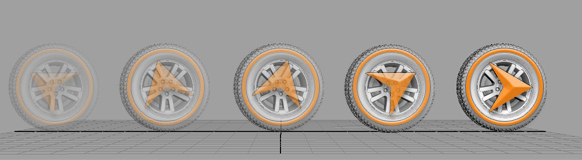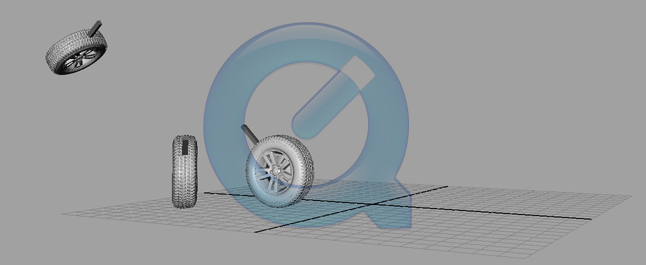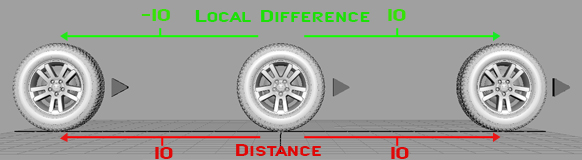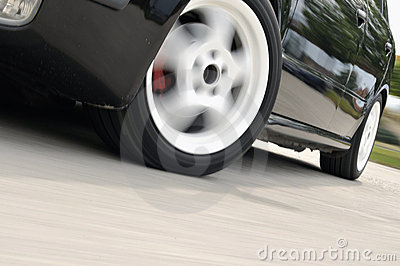Calculating Wheel Rotation
Kiel Figgins - 3dFiggins.comShort Version

• Don't just find the distance traveled, find the local difference between two frames
• Use the circumference of the wheel and difference value in this equation to bake out a channel for the rotation frame by frame
•      float \$finAmount = ( (-((\$theDist / \$userCircum) * 360)) + \$difValue);
• Maya MEL script to do this for you: kfWheelRotation.mel
• Tips/Tricks and Final Thoughts for streamlining the process

Introduction
The Process
1. Calculating the orientation distance traveled
2. Finding the Circumference of the wheel
3. Using the Distance traveled value to drive wheel rotation
MEL Script
Tips and Tricks
Final Thoughts

Introduction

The most common approach to calculating wheel rotation, and also similar to the one I use, is a postscript that bakes rotation per frame based on the distance traveled from the last frame. However, this process has certain drawbacks, for example the wheel going straight up (such as being raised on an elevator) or fishtailing sideways. In these instances, the wheel is not moving forward, so would not need to rotate. The solution that I've found is a bit more involved than judging distance per frame, but rather the difference per frame.Fig. 1 - Wheel Rotation Example Movie (4mb)

The Process

1. Calculating the orientation distance traveled
Once your wheel is animated traveling through space, you create a duplicate of your wheel on the current frame and another one frame ahead. Once these two duplicates are created, parent the first duplicate wheel under the second. The resulting tz, or corresponding translate axis, value of the first duplicated wheel is the difference in distance that will be used to rotate the wheel. Using this approach, you can determine the distance traveled with appropriate direction as well as reverse.Fig. 2 - Distance vs Difference

Though this process may seem confusing, you can see it working in this example:
```-Make a wheel (cylinder) at origin (name it wheelOrg)
-Duplicate wheelOrg, name it wheelDiff

(Forward motion)
-Set wheelDiff.tz to 10
-Parent wheelOrg to wheelDiff
-The tz of wheelOrg is now -10

(Reverse motion)
-unparent wheelOrg from wheelDiff
-Set tz of wheelOrg to -10
-Parent wheelOrg to wheelDiff
-The tz of wheelOrg is 10
```

From this example, if you were only using the distance between the two points (or frames), you'd get the same value of 10. However, using the 'difference' method, you get a value that will indicate direction changes. Repeating this example by plugging in a value 10 in Translate Y of wheelDiff, you'll see that even though the wheel moved the rotation would not be calculated because it didn't move on its 'forward' axis.

2. Finding the Circumference of the wheel

Finding the circumference of a wheel is pretty straight forward.
```-Create a default polyClyinder
-Align the cylinder to the wheel, but don't scale it, only use position and rotation
-Using the INPUTS of polyCylinder1, adjust the radius amount until they're the same size
-Use that radius amount in the following equation to find Circumference:

c = 2 * 3.14159 * radius Amount

```Fig. 3 - Finding the Circumference with a proxy cylinder

3. Using the Distance traveled value to drive wheel rotation

Having calculated the proper distance traveled, you'll need gather the current rotation value of the wheel:
```float \$curAmount = `getAttr Wheel_Spin.rx`;
```

Now that you have the circumference, proper distance amount, and current rotation value, you can plug them into this equation:
```float \$finAmount = ( (-((\$theDist / \$userCircum) * 360)) + \$curAmount);
```

What this equation is saying is: take the distance traveled, divide it by the circumference, multiple it by 360 (to put it in degrees), inverse it, then add to the existing rotation amount. From here you'd set the \$finAmount to the rotation channel, then set a keyframe.
```setAttr Wheel_Spin.rx \$finAmount;
setKeyframe Wheel_Spin.rx;
```

After that, you'd loop through all the frames, repeating this process. Since that would be pretty labor intensive, here's a MEL script to do it for you.

MEL ScriptFig. 4 - Wheel Rotation MEL Script

kfWheelRotation.mel

To use the script:
```-Window > General Editors > Script Editor
-In the Script Editor, File > Source Script
-then run the following command:

kfWheelRotation();
```

Once the window opens, define the wheel object by selecting it, then pressing the '<' button. Fill in the remaining fields, set the timeline to desired length and hit the 'Calculate' button. If all works out correctly, the script should run through the timeline and bake out the values.

Tips and Tricks

1. If you plan to use this process for a car, you'll need to calculate a new value per wheel, as each wheel travels slightly different amounts per frame. A slight modification to the script would allow you to do this without having to go through the timeline for each wheel.

2. Finding the circumference can be tedious and unnecessary to do each time you wish to run the script. If you find the circumference once, create a new channel on the wheel control and put the circumference value on that channel. Again, a small modification to the script to look for that channel and use the value could save you additional time in streamlining the process.

3. When setting up a rig for a car, keep autoWheelSpin (or whatever channel you wish to drive the wheel rotation) as a separate channel. This would allow an Animator to override or remove it if the shot calls for it. The creation of 2 channels: autoWheelSpin and manualWheelSpin would be added together to allow Animators explicit control over the wheel.

4. For this process to work, you'll need the wheel transform to actual move per frame, not deform, as the calculation is still based off the pivot. If wheel is deforming, use the wheel control instead to find the distance.

Final Thoughts

Overall, calculating realistic wheel rotation is tricky, since a wheel has roughly four states: stopped, rolling, speeding up and cruising. Obviously, if the car is stopped, finding the rotation isn't necessary. Speeding up and cruising rotation values may need to be adjusted regardless, if the blur on the wheel starts to 'wagon wheel' (spinning so fast the motion blur makes it look like its spinning slowly backwards) and becomes distracting. So that leaves rolling, a fairly rare shot in production. If you have a rolling shot, this process would be used.Fig. 5 - Example of motion blur causing a 'wagon wheel' effect

Another factor is speed and scale. Speed is not always accurate in 3d, usually because the scene or assets aren't built to real world units or travel realistic distances.

With all said and done, if your animation requires a precise rotation, the above approach should get you there. If it doesn't, than eyeballing the rotation values should be enough to keep you moving forward.